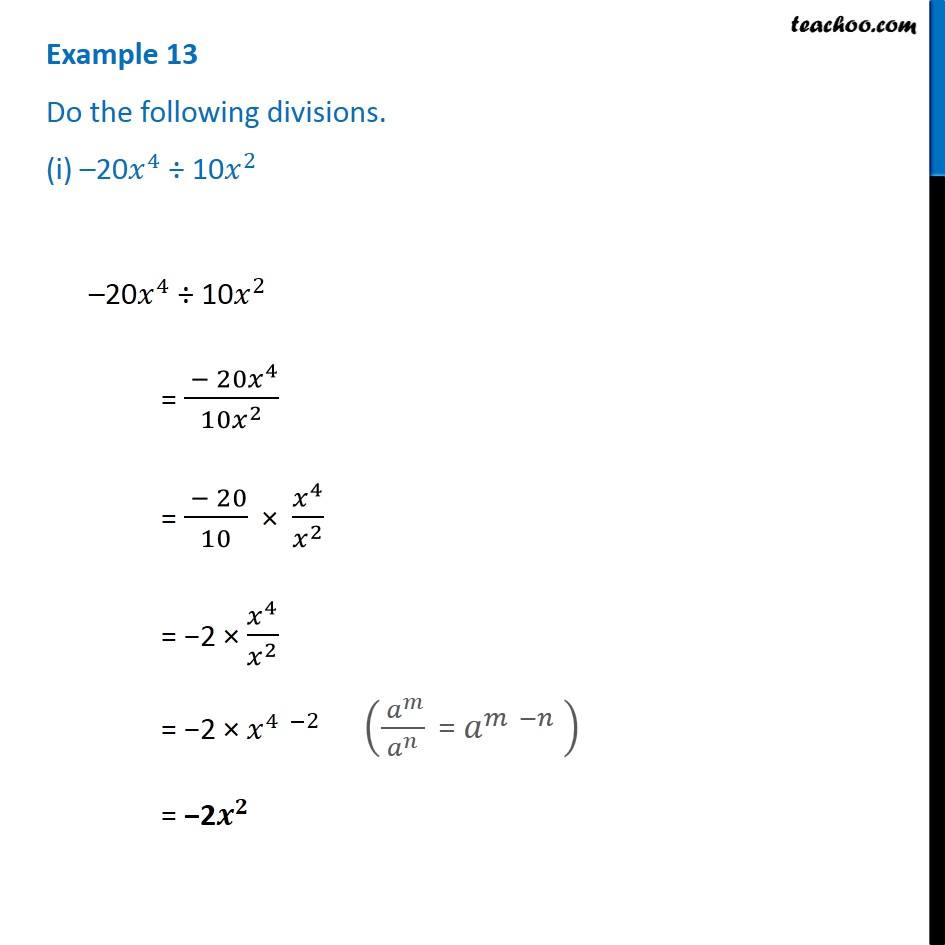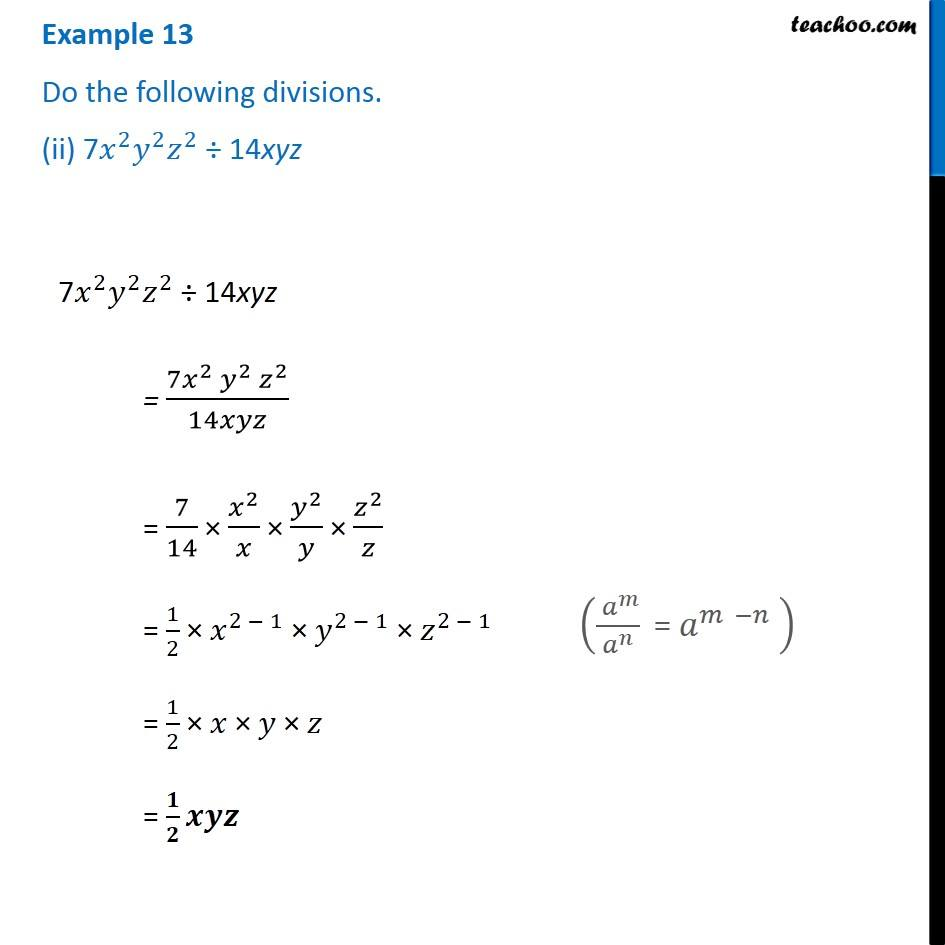Subscribe to our Youtube Channel - https://you.tube/teachoo

1. Chapter 14 Class 8 Factorisation
2. Serial order wise
3. Examples

Transcript

Example 13 Do the following divisions. (i) –20𝑥^4 ÷ 10𝑥^2 –20𝑥^4 ÷ 10𝑥^2 = ( − 20𝑥^4)/(10𝑥^2 ) = ( − 20)/10 × 𝑥^4/𝑥^2 = −2 × 𝑥^4/𝑥^2 = −2 × 𝑥^(4 −2) = −2𝒙^𝟐 (( 𝑎^𝑚)/𝑎^𝑛 " = " 𝑎^(𝑚 −𝑛) " " ) Example 13 Do the following divisions. (ii) 7𝑥^2 𝑦^2 𝑧^2 ÷ 14xyz 7𝑥^2 𝑦^2 𝑧^2 ÷ 14xyz = (7𝑥^2 𝑦^2 𝑧^2)/14𝑥𝑦𝑧 = 7/14 × 𝑥^2/𝑥 × 𝑦^2/𝑦 × 𝑧^2/𝑧 = 1/2 × 𝑥^(2 − 1) × 𝑦^(2 − 1) × 𝑧^(2 − 1) = 1/2 × 𝑥 × 𝑦 × 𝑧 = 𝟏/𝟐 𝒙𝒚𝒛 (( 𝑎^𝑚)/𝑎^𝑛 " = " 𝑎^(𝑚 −𝑛) " " )

Examples